# System of equations - 7th grade (12y) - math problems

#### Number of problems found: 178

• HostelStudents are accommodated in 22 rooms. Rooms were 4 and 6 bed. How many rooms in which type occupied 106 children there?
• Addends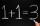Number 839 divide into the two addends that the first was 17 greater than 60% of the second. Determine these addends.
• Coffee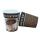Coffee from the machine in the cup cost 28 cents. Coffee is 20 cents more expensive than the cup. How much is the cup?
• Triangle anglesThe angles α, β, γ in triangle ABC are in the ratio 6:2:6. Calculate size of angles.
• Magic numberThe number 135 split to two addends so that one addend was 30 greater than 2/5 the addend.
• FamilySon is 3/5 smaller than the mother. Mother is 99 cm higher than the son. How tall is the mother?
• Two numbersThe sum of two numbers is 1. Identify this two numbers if you know that the half of first is equal to the third of second number.
• Age questionJano is three times older than Zdeno. Zdeno is 12 years younger than Janko. How old is Jano, and how old is Zdeno?
• Pair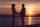They have together 39 years - he has 2 times as she was when he was equal as she has today ....
• Jam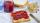Jar with jam weighs 800 grams. Jam weighs three times as much as the empty glass. What is the weight of jam that is in the glass?
• Bottle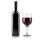Bottle with wine costs 58 USD. Wine is 27 USD more expensive than the empty bottle. How much is the bottle itself?
• Bases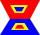The length of the bases trapezium are in ratio 4:5. Length of midline is 15. How long are the bases of a trapezoid?
• Revenue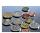Mr. Robert and Mr. Norbert get together € 1400. The revenue split in the ratio of 4:3, depending upon each work. How many got each of them?
• Tippler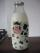Bottle with cork cost 8.8 Eur. The bottle is 0.8 euros more expensive than cork. How much is a bottle and the cork?
• Brothers and sistersLenka has as many brothers as sisters. Her brothers have twice as many sisters as brothers. How many brothers and sisters are in the family?
• Blueberries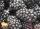Miko and Anton have a total of 1,580 blueberries. Miko and Anton have them in the ratio 2: 3. Determine how much each of them has.
• Ratio three numbers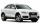Three numbers SUV are in the ratio 1:2:3. Their sum is 24. Find this numbers and write their add and sum.
• Internal anglesOne internal angle of the triangle JAR is 25 degrees. The difference is the size of the two others is 15°. Identify the size of these angles.
• Rabbit family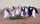Rabbit family ate 32 pieces of carrots, small one ate 6 pieces, dad 5 more than the mother. How much ate mom?
• Ski lessons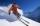On the ski course leaves 80 pupils. They live in triple and quadruple rooms. Count if rooms is the 23. How many are triple and quadruple rooms?

Do you have an interesting mathematical word problem that you can't solve it? Submit a math problem, and we can try to solve it.

We will send a solution to your e-mail address. Solved examples are also published here. Please enter the e-mail correctly and check whether you don't have a full mailbox.

Please do not submit problems from current active competitions such as Mathematical Olympiad, correspondence seminars etc...

Do you have a system of equations and looking for calculator system of linear equations? System of equations - math problems. Examples for 7th grade (seventh).